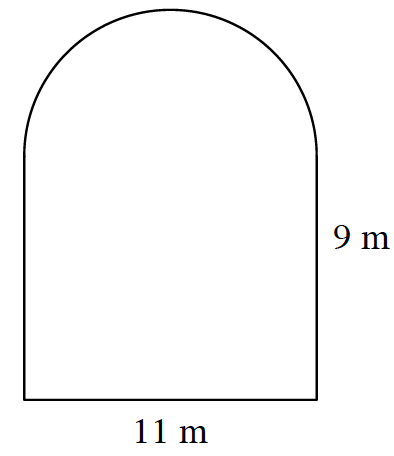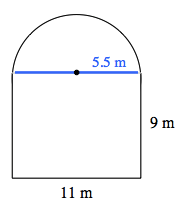### Home > CCAA > Chapter 9 Unit 8 > Lesson CC2: 9.1.3 > Problem9-45

9-45.

The shape at right is composed of a rectangle and a semi‑circle (half of a circle).1. Find the perimeter.The perimeter of a semi-circle is half of the circumference of a circle.

If the semi-circle were made into a complete circle, its circumference would be $11\pi$.

$46.27$ meters

2. Find the area.

Find the area of the semi-circle and the area of the rectangle separately, then add them together to find the total area.